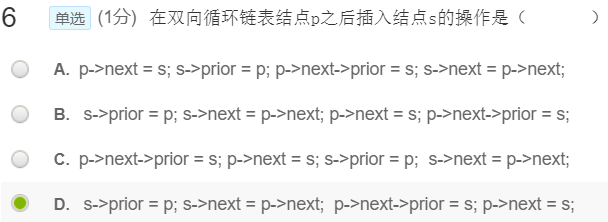• 双向链表 指针 数据静态变量 静态方法 vc6.0的编程环境，用类实现双向链表
• 最近看《零基础学java》，数据结构中看到双向链表，说每个链表元素都有两个指针属性，一个是previous指向上一个元素本身，另一个是next执行下一个元素本身。可双向链表的结构图却是相反的。问具体是什么情况
• 用一个指针实现双向链表这个东西除了面试中能够用到，其他地方哪里会用到，这个我也不知道。希望知道的人能够评论中说下。 下面直接给出代码 typedef struct _Q1LinkNode { int data; unsigned long ...
用一个指针实现双向链表这个东西除了在面试中能够用到，其他地方哪里会用到，这个我也不知道。希望知道的人能够在评论中说下。

下面直接给出代码

typedef struct _Q1LinkNode
{
int data;

// 创建一个节点
{
node->data = data;
return node;
}
// 添加一个节点
{
unsigned long l = 0, prior = 0;
while (1) {
if (prior == 0) {
p->link = l ^ (unsigned long)node;

l = (unsigned long)p;
return node;
}
l = (unsigned long)p;

}
}
// 打印节点
{
unsigned long l = 0 , prior = 0;
printf("%d ",p->data);
while (1) {
if (prior == 0) {
break;
}
l = (unsigned long)p;
printf("%d ",p->data);
}
}

Q1LinkNode *h1 = createQ1LinkNode(78);

printQ1Node(h1);
printQ1Node(h2);


展开全文c语言
• 在双向链表存储结构中，删除p所指的结点时须修改指针()【MOOC选择题】展开全文数据结构
• 在双向链表存储结构中，删除p所指的结点时需修改指针的操作为 p-&gt;next-&gt;prior=p-&gt;prior; p-&gt;prior-&gt;next=p-&gt;next; p-&gt;next=p-&gt;next-&gt;next;p-&...
在双向链表存储结构中，删除p所指的结点时需修改指针的操作为 p->next->prior=p->prior; p->prior->next=p->next; p->next=p->next->next;p->next->prior=p; p->prior->next=p;p->prior=p->prior->prior; p->prior=p->next->next;p->next=p->prior->prior;
答案：A
展开全文• 数据结构c++双向链表（首尾指针） 插入操作 #pragma once #include <iostream> using namespace std; template <class T> class DoubleLinkList; template <class T> class Node { public: ...
数据结构c++双向链表（首尾指针）
插入操作#pragma once
#include <iostream>

using namespace std;

template <class T>

template <class T>
class Node
{
public:
Node(T data)
{
this->data = data;
pre = nullptr;
next = nullptr;
}

friend ostream& operator<<(ostream& os, Node<T>* node)
{
return os << node->data;
}
private:
T data;

Node<T>	*pre;		//上一节点

Node<T> *next;		//下一节点
};

template <class T>
{
public:
{
first = last = new Node<T>(-1);
size = 0;
}

bool is_empyt()
{
if (size == 0)
return true;
return false;
}

void Clear()
{
if (is_empyt())
return;
auto p = first->next;
Node<T>* temp = nullptr;
while (p!=last)
{
temp = p;
delete temp;
p = p->next;
}
last = first;		//重置末节点
size = 0;
}

void show_list()
{
if (is_empyt())
return;
auto p = first->next;
while (p != nullptr)
{
cout << p;
p = p->next;
}
}

Node<T>* get_node(int index)
{
if (is_empyt())
return nullptr;
if (index<0 || index>size)
return nullptr;
auto p = first->next;
for (int i=0;i<size;++i)
{
if(i==index)
break;
p = p->next;
}
return p;
}

void insert_index(T data, int index)
{
if (index<0 || index>size)
return;
auto node = new Node<T>(data);
if (size == 0)
{
node->pre = first;
first->next = node;
last = node;
}
else if (index == 0)
{
node->pre = first;			//把首节点赋值给新节点的前驱
node->next = first->next;	//将第一个节点赋值给新节点的后驱
first->next->pre = node;	//将新节点赋值给第一个节点的前驱
first->next = node;			//将新节点赋值给首节点的后驱
}
else if(index == size)
{
last->next = node;
node->pre = last;
last = node;
}
else
{
auto pre_node = get_node(index-1);		//当前节点的上一节点
node->pre = pre_node;
node->next = pre_node->next;
pre_node->next->pre = node;
pre_node->next = node;
}
++size;
}

void push_back(T data)
{
insert_index(data, size);
}

void push_front(T data)
{
insert_index(data, 0);
}

T pop_index(int index)
{
if (index<0 || index>size-1)
return -1;
Node<T>* node = nullptr;
if (index == 0)		//首
{
node = first->next;
first->next = node->next;
node->pre = first;
if (size == 1)
last = first;
}
else if (index == size-1)		//尾
{
node = last;
last = last->pre;
last->next = nullptr;
}
else	//中
{
node = get_node(index);		//当前位置节点
node->pre->next = node->next;
node->next->pre = node->pre;
}
T data = node->data;
delete node;
--size;
return data;
}

T pop_back()
{
return pop_index(size-1);
}

T pop_front()
{
return pop_index(0);
}
private:
Node<T>* first;

Node<T>* last;

int size;
};


展开全文c++ 数据结构
• 指针双向链表逻辑结构单指针双向链表则需要采用异或链表的方式，下图是一个具有五个节点的双向链表的逻辑结构示意图，没有头结点。其中每个节点的后半部分表示指针域，存储了它的前驱节点的指针与后继借点的指针的...c语言
• 双向链表的结点时结构体，有数据本体，指向一元素的指针prev以及指向后一元素的指针next组成。这些结构体通过指针构成一个链表，就形成了双向链表。 struct node{ int key; node *prev,*next;//注意不是node...list
• 双向链表 定义 ... 双向链表的结点包括一个数据域和两个指针域,后继指针指针双向链表的后继链和继首尾相连,为空双向链表,又称双向循环链表 双向链表是一种对称结构,提供了向前搜索和向链表 单链表 结构 线性表
• 数学基础 离散数学中的异或运算a⊕b，具有以下性质： a⊕b = b⊕a a⊕a = 0 ...a⊕(a⊕b) = (a⊕a)⊕b = b ...单指针双向链表的逻辑结构 下图是一个具有五个节点的双向链表的逻辑结构示意图，没有头结点。 ...
• ## 链表指针

千次阅读 2014-03-01 10:36:15
前言：学习链表的基础是你要对动态分配内存和结构体指针有所了解。 一、什么是链表链表就是一种动态分配内存的数据结构。 二、链表的作用或者说好处。 1.链表可以有效的节省内存。 三、链表的基本操作 1.定义： ...移动的小球
• 双向链表：单向链表只能向着一个方向遍历链表节点，而节点指针域中增加了指针双向链表，则可以向着两个方向遍历节点。这使得双向链表也可以任何一个节点遍历整个链表。 function DoublyLinkedList() { ...
• 指针异或运算实战二、异或指针双向链表的实现 一、异或运算的基本知识 1. 什么是异或运算 假设二进制数10跟二进制数01进行异或运算的时候，即10 ^ 01，从右往左依次进行运算，相同情况的异或结果为0，否则为1。...
• 那么本文将介绍一下另一种特殊的链表结构，叫做 双向链表。 顾名思义，普通的链表都是从 head 开始往后遍历结构内的元素，那么双向链表就是既可以从头开始遍历，又可以从结构的末尾开始遍历。 上一篇文章的跳转链接...数据结构 算法 javascript 前端
• 已知不带头结点的双向链表第一个节点的指针为list，链节点除了数据域和分别指向该结点的前驱结点和后继结点的指针域外，还设置记录该节点访问次数频度域freq(初始值为0)，请设计一算法LOCATE(list,x),该算法的功能是...
• 单链表和双链表 链表结构： 优点： 1、程序中使用数组之前，必须事先知道数组的大小，增加数组的大小...由于在链表中，仅仅只有头节点和尾节点是可见的，因此要想查找某个节点，必须从头节点或尾节点一路找下去，时间单链表 数据结构 java
• 双向链表也叫双链表，是链表的一种，它的每个数据结点中都有两个指针，分别指向直接后继和直接前驱。所以，从双向链表中的任意一个结点开始，都可以很方便地访问它的前驱结点和后继结点。一般我们都构造双向循环链表...
• 双向链表： 就是有双向指针 即 双向的链域 链结点的结构： ┌────┬────┬────────┐ │data│next│previous│ └────┴────┴────────┘ 双向链表不必是双端链表(持有对最后一个...数据结构 链表
• ## JAVA双向链表

千次阅读 2019-04-19 16:29:58
双向链表的每一个结点都有一条指向其后继结点的next指针和一条指向其结点的pre指针双向链表的插入图示： 双向链表的删除图示： import java.io.IOException; import java.util.Scanner; class Node{ public...java
• 添加（默认添加到双向链表的最后） （1）.先找到双向链表的最后这个节点（temp） （2）.temp.next = newHeroNode （3）.newHeroNode.pre = temp; 修改思路和单链表一样 删除 （1）.以为是双向链表，因此，我们可以...java
• 对循环双链表实现下述功能： void meau(); //菜单函数 void Initlist(List *list); //初始化 void show(List *list); //打印链表内容 bool Push_back(List *list,ElemType x); //尾插法 b...